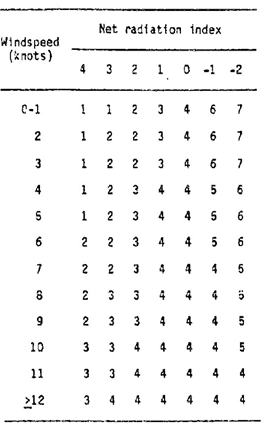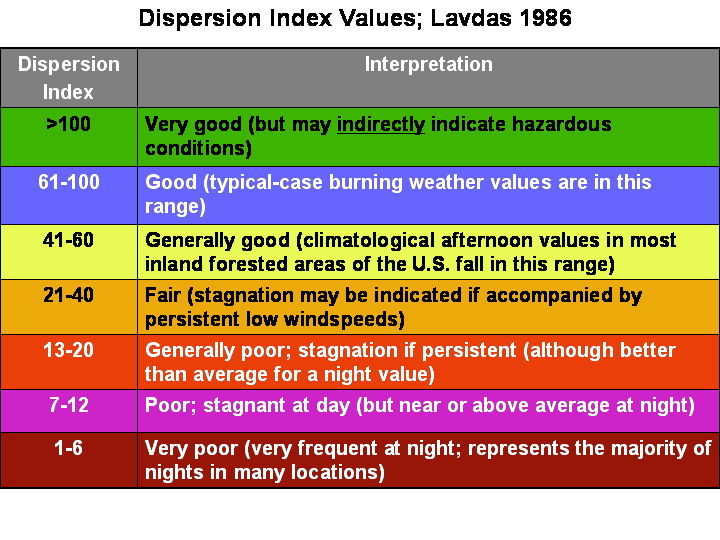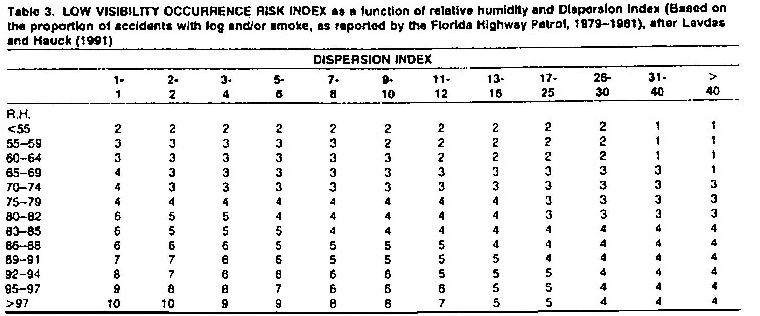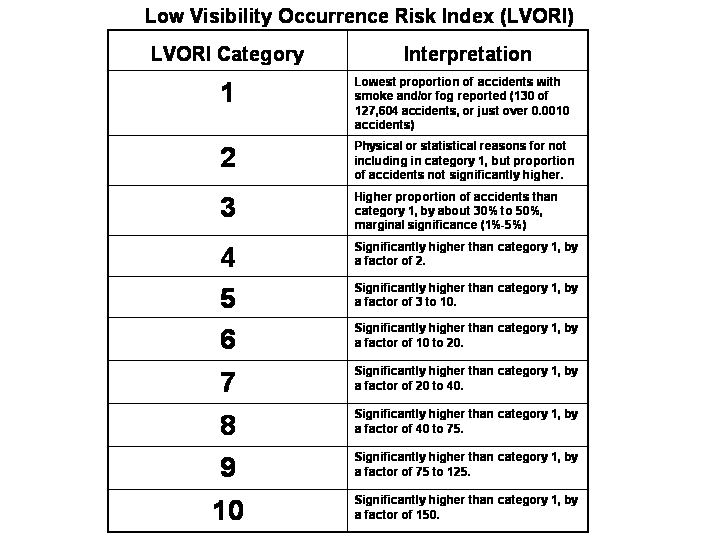Basic Guide to Calculating Atmospheric Dispersion Index (ADI, after Lavdas)
1.    ADI is derived from mixing height and stability class. The NWS Tampa ADI software uses mixing heights from the forecast
grids. Stability class is derived from the Net Radiation Index (NRI), and surface wind speed from the forecast grids. NRI
can be obtained using the list of cloud and ceiling rules below. The software uses a model ceiling tool, determining ceilings
from GFS model moisture. Notice that for daytime (rule list III), an Insolation Class (IC) number must be determined, using
solar elevation angles. The IC is then adjusted by the daytime rules, yielding an NRI.
I. If the total opaque cloud cover is 10/10 and the ceiling height is <7,000 ft, use net radiation index equal to 0 (whether
day or night).

II. For nighttime

A. If total opaque cloud cover =< 4/10, use net radiation index equal to -2.

B. If total opaque cloud cover >4/10, use net radiation index equal to -1. (Note that this will not apply for cases of
10/10 coverage when ceiling < 7,000 ft, since such cases are determined by step I, above).

III. For daytime:

A. Determine the insolation class number as a function of solar elevation angle according to the following table:

`             Solar Elevation Angle (d)	Insolation Class Number`
```                    60 < d                                4

35 < d =< 60                          3

15 < d =< 35                          2

d =< 15                          1```
The ADI software from Tampa uses Julian dates for the beginning and end dates when the noon sun elevation angle will
be above 60 degrees, and assumes the rest of the year will have noon sun angles between 35 degrees and 60 degrees.
Since noon sun angles in winter at GSP are below 35 degrees, but always above 15 degrees, the IC test was modified
to include Julian dates for lower winter sun angles. If a site has winter noon sun angles below 15 degrees, a further line
of code would need to be added to the IC test with the beginning and ending Julian dates of those angles. Winter sun
angles can be determined from the NOAA Solar Position Calculator, at https://www.esrl.noaa.gov/gmd/grad/solcalc/.
B. If the total opaque cloud cover =< 5/10, the net radiation index is equal to the insolation class number.

C. If the total opaque cloud cover > 5/10, modify the insolation class number by following these six steps:

1. Ceiling height less than 7,000 ft, subtract 2.

2. Ceiling height >= 7,000 ft but < 16,000 ft, subtract 1.

3. Total opaque cloud cover equal to 10/10, subtract 1. (This will only apply to ceilings >= 7,000 ft because cases
with 10/10 coverage with ceiling < 7,000 ft are determined by step I, above.)

4. If neither steps 1 and 2 nor 3 immediately above are applicable, assume the modified insolation class number is equal to the insolation class number.

5. If the modified insolation class number is less than 1, let it equal 1.

6. Set the net radiation index equal to the modified insolation class number.

2. Using the NRI, surface wind speed, and the table below, the stability class can be derived.3. Use the stability index (step 2), forecast mixing height, and forecast transport wind to derive the dispersion index (ADI) from the chart below. Notice that the stability class is labeled for day or night, and that there is a different class number four for both day and night.
4. Interpretation of the ADI is listed in the table below.Basic Guide to Calculating Low Visibility Occurrence Risk Index (LVORI, after Lavdas and Achtemeier)
1. LVORI is calculated from the ADI and relative humidity, both of which are obtained from the forecast grids by the NWS LVORI software. The figure below shows the relationship between relative humidity, dispersion index, and smoke or fog related traffic accidents.
2. The table below can be used to obtain LVORI from ADI and relative humidity. The bottom of the table is a key to LVORI values and their corresponding relationship to traffic accident.3. The last table shows the relationship between LVORI and low visibility reports.
4. When visibility reductions with advection fog occur, higher wind speeds will yield a higher ADI, and thus a lower LVORI. Notice in the figure below, as wind speeds increase, the relative frequency of advection fog dwarfs that of radiation fog.